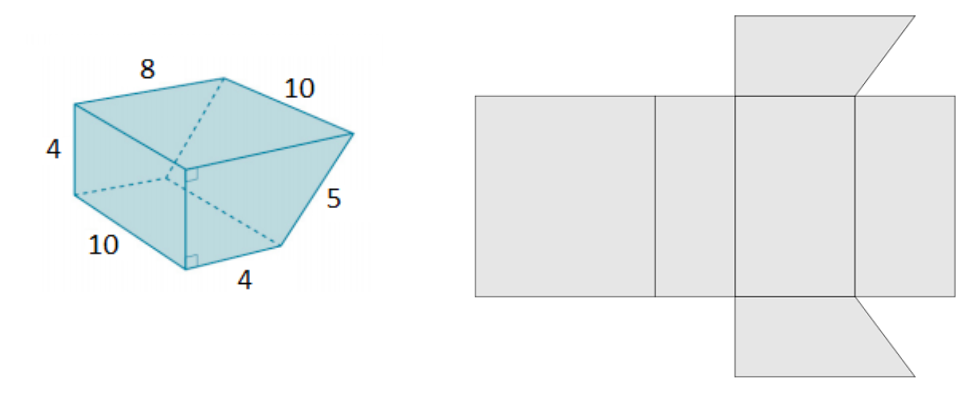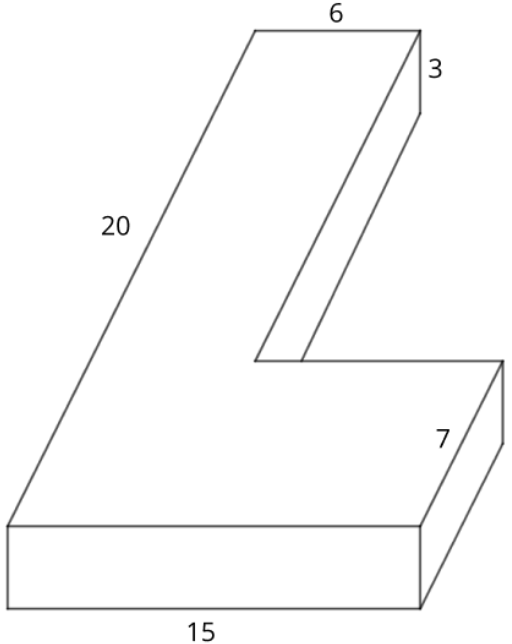# Geometry

## Objective

Find the surface area of right prisms.

## Common Core Standards

### Core Standards

?

• 7.G.B.6 — Solve real-world and mathematical problems involving area, volume and surface area of two- and three-dimensional objects composed of triangles, quadrilaterals, polygons, cubes, and right prisms.

?

• 6.G.A.4

## Criteria for Success

?

1. Use a net of a three-dimensional figure to determine the surface area.
2. Find efficient ways to determine surface area of right prisms by analyzing the structure of the shapes and their nets (MP.7).

## Tips for Teachers

?

Lessons 17 and 18 engage students in finding the surface area of prisms and pyramids. Students leverage their skills with numerical expressions to organize and represent their work in finding the surface area. Lesson 17 focuses on prisms and Lesson 18 focuses on pyramids. In Lesson 21, students will revisit surface area as it compares to volume in real-world contexts.

#### Remote Learning Guidance

If you need to adapt or shorten this lesson for remote learning, we suggest prioritizing Anchor Problem 1 (benefits from worked example). Find more guidance on adapting our math curriculum for remote learning here.

#### Fishtank Plus

• Problem Set
• Student Handout Editor
• Vocabulary Package

## Anchor Problems

?

### Problem 1

A trapezoidal prism and its net are shown below.1. Complete the net by labeling all of the dimensions.
2. Find the surface area of the prism.
3. What are the dimensions of the long rectangle, composed of 4 smaller rectangles, in the net? How are the dimensions related to the trapezoid base?

### Problem 2

Imagine that a piece of the rectangular prism is removed. Determine the surface area of both pieces.#### References

EngageNY Mathematics Grade 7 Mathematics > Module 6 > Topic D > Lesson 23Example 1b

Grade 7 Mathematics > Module 6 > Topic D > Lesson 23 of the New York State Common Core Mathematics Curriculum from EngageNY and Great Minds. © 2015 Great Minds. Licensed by EngageNY of the New York State Education Department under the CC BY-NC-SA 3.0 US license. Accessed Dec. 2, 2016, 5:15 p.m..

## Problem Set

?The following resources include problems and activities aligned to the objective of the lesson that can be used to create your own problem set.